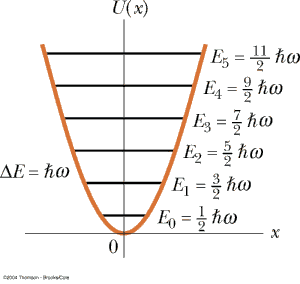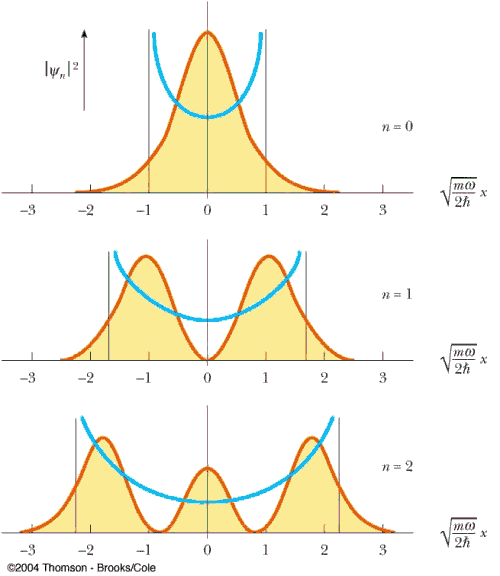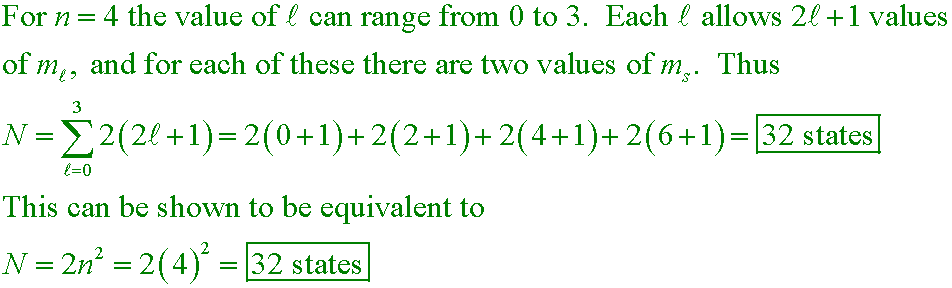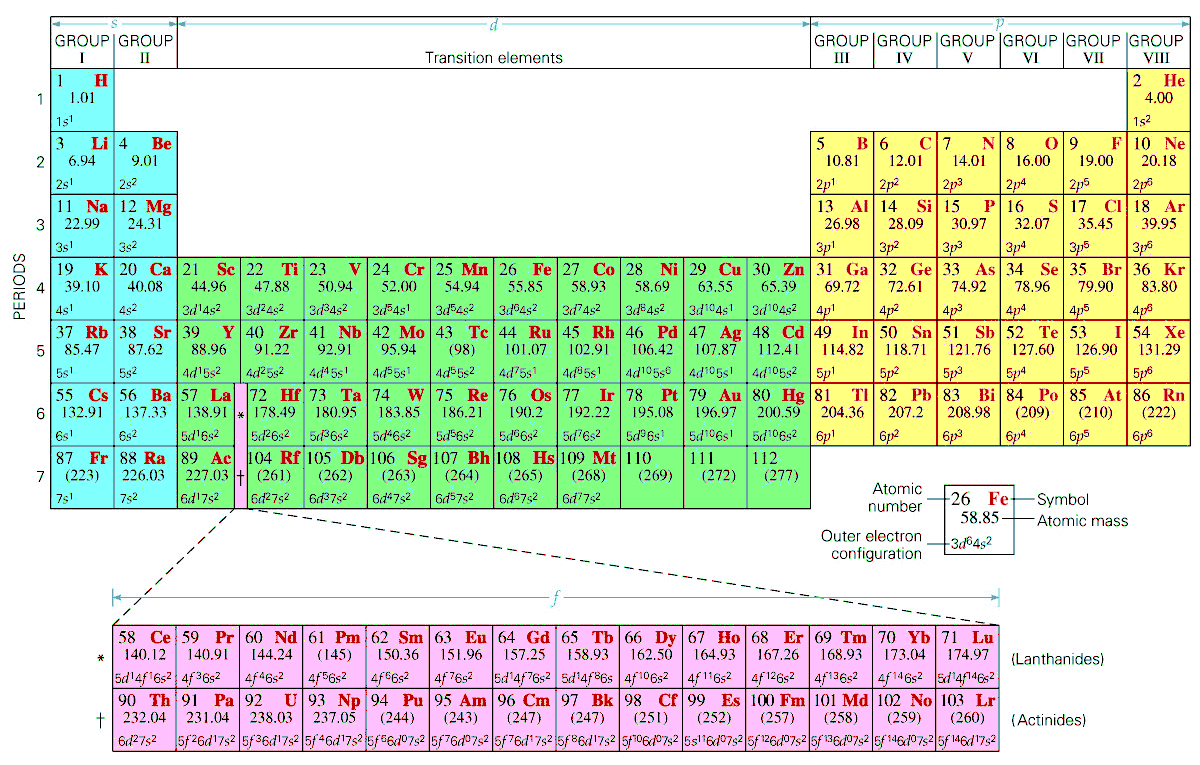﻿ Lecture 44 Summary
• The finite box
• Tunneling
• The simple harmonic oscillator• The Simple Harmonic Oscillator
• problem 7.51
• energy levels
• example problem
• turning points
• probability densities
• orthonormal basis setklm
How many different states are possible for an electron whose principal quantum number is n = 4? A. 8            B. 18            C. 32            D. 50
Answer

sj6 42.38
What is the probable electronic configuration of a neutral atom of element 110?
A. [Rn] 5f 14 6d 2 6f 6
B. [Rn] 5f 14 6d 0 6f 8
C. [Rn] 5f 14 6d 8 7s2
D. [Rn] 5f 16 6d 6 7s2
Answer

C. 32C. [Rn] 5f 14 6d 8 7s2
Use the diagrams above to determine that the 7s subshell will fill up before the 5f or 6d subshells. Along the block of elements from 104 to 112 the 6d subshell is being filled. There is in reality an anomaly here and the actual observed configuration is 5f 14 6d 9 7s1.